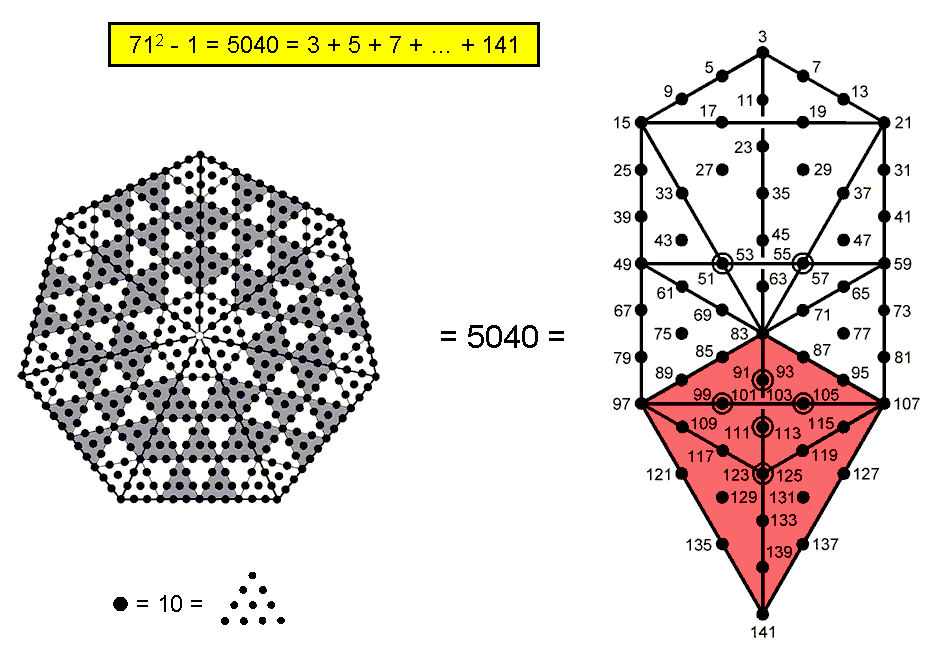<< Previous    1...   13  14    16  17  ...56    Next >>

#15 The heptagon & Tree of Life embody the superstring structural parameter 504There are 504 yods surrounding the centre of a heptagon whose sectors are 2nd-order tetractyses. If the Decad (10) is assigned to each yod, the heptagon generates the number 5040. As

712 − 1 = 3 + 5 + 7 + ... + 141 = 5040,

this number is the sum of the first 70 odd integers after 1 that can be assigned to the 70 yods in the Tree of Life whose 16 triangles are tetractyses. As 5040 = 70×72, the average of these odd integers is 72, which is the number of yods per sector of the heptagon. 72 is the number of Chesed, the first Sephirah of Construction.

As 5040 = 3×1680, this number is the number of circular turns in the three major whorls of the UPA, the basic unit of matter described by Besant & Leadbeater (see here). Each whorl revolves five times about the axis of spin of the UPA. The number 504 represents therefore the number of turns in a half-revolution of all three major whorls.

The Lower Face of the Tree of Life is the light red kite-shape in the Tree of Life shown opposite whose vertices are located at Tiphareth, Netzach, Hod & Malkuth (but note that this shape is only a cross-section, because the Tree of Life is 3-dimensional, so that the Lower Face also contains Yesod and the Paths linking it to the four other Sephiroth). The Lower Face consists of 30 yods, so that the region of the Tree of Life that is outside the kite contains 40 yods. As

412 − 1 = 3 + 5 + 7 + ... + 81 = 1680,

the number 1680 is the sum of the first 40 odd integers after 1 that can be assigned to these 40 yods. This means that the sum of the next 30 odd integers: 83, 85, .... 141 that can be assigned to the 30 yods in the Lower Face is 2×1680 = 3360. The region outside the Lower Face embodies the number of turns in a helical whorl, the Lower Face embodies the number of turns in one revolution of all ten whorls and the Tree of Life itself embodies the number of turns in the three major whorls. Its geometry is such that the arithmetic division of the number 5040 into 1680 and 3360 corresponds to the distinction between the Lower Face and the remainder of the Tree of Life. This remarkable harmony between sacred geometry and odd numbers cannot be due to chance, especially as it manifests in the micro-psi account by Besant & Leadbeater of what they claimed is the basic constituent of matter. How much 'coincidence' can the die-hard sceptic tolerate before he has to admit that his persistent explanation in such terms is so miraculously improbable as to be rationally indefensible?

 << Previous    1...   13  14    16  17  ...56    Next >>

Home